Science
Our World |

# 10 Notable Numbers

fact checked by Alex Hanton

Numbers effect most aspects of our lives daily. This is a list of 10 notable numbers.

10. -273.15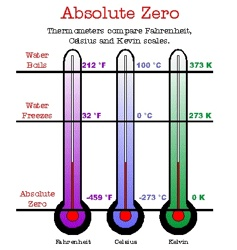This is the temperature (in centigrade) known as absolute zero (0 Kelvin) which describes a theoretical system that neither emits nor absorbs energy. It is the point at which particles have a minimum energy, determined by quantum mechanical effects, which is called the zero-point energy. Absolute zero is also precisely equivalent to 0 °R on the Rankine scale (also a thermodynamic temperature scale), and –459.67 °F on the Fahrenheit scale. It is not possible to cool any substance to 0 K, but scientists have made great advancements in achieving temperatures close to absolute zero, where matter exhibits odd quantum effects such as superconductivity and superfluidity.

If you’re going to survive an extreme winter, you might need a Magnesium Fire Starter at Amazon.com!

9. 31337If you don’t know what this one means, the number does not apply to you. If you are not 31337 and want to know how to become 31337, Wikipedia has a good article on it here.

8. -40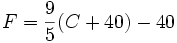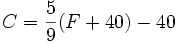This number is unique in temperature readings; it is unique because -40 is the only point at which the fahrenheit and centigrade scales meet. -40F is the same as -40C.

7. 3888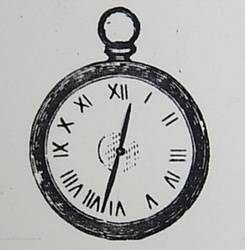This is the largest number representable with standard Roman numeral notation. In Roman numerals it appears: MMMDCCCLXXXVIII.

6. 666666 is most famous as the number of the beast in the biblical book of the Apocalypse. It is also the former number of Route 491, a brand of cough syrup, and forms part of the name of 666 Desdemona, a minor planet in the asteroid belt. A person with a fear of this number has hexakosioihexekontahexaphobia.

Click here to access 40,000 movies and TV shows with Amazon Prime Instant Video – 30 day free trial at Amazon.com!

5. 911Before the terrorist attacks on the US, this number was also attached to a type of porsche and a trojan asteroid that orbits the sun. It is, of course, also the US, Canadian, and Argentinean emergency services telephone number.

4. Graham’s Number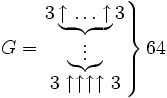Graham’s number is the largest number that has been used in a serious mathematical proof. It is too large to be written in scientific notation and is much larger than a googol, googolplex, and is even larger than Moser’s number, another very large number. Using Knuth’s up-arrow notation, Graham’s Number (G) can be depicted as in the image above.

3. e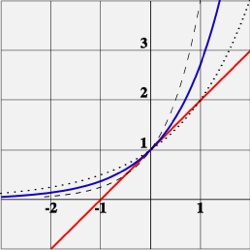The number e, sometimes called Euler’s number or Napier’s constant, is one of the most important numbers in mathematics. Bernoulli discovered this number when studying a problem of compounding interest. Simply put, an account that starts at \$1, and yields (1+R) dollars at simple interest, will yield eR dollars with continuous compounding. The number e itself also has applications to probability theory, where it arises in a way not obviously related to exponential growth. Suppose that a gambler plays a slot machine with a one in n probability and plays it n times. Then, for large n (such as a million) the probability that the gambler will win nothing at all is (approximately) 1/e. e looks like this to 20 decimal places: 2.71828 18284 59045 23536…

2. Pi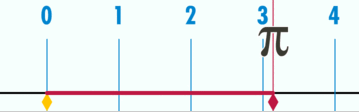Pi or ? is the ratio of a circle’s circumference to its diameter in Euclidean geometry, approximately 3.14159. Pi is a mathematical constant and a transcendental (and therefore irrational) real number, with many uses in mathematics, physics, and engineering. It is also known as Archimedes’ constant.

1. 1.6180339887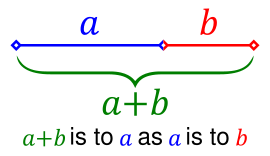This is the Golden Ratio. In mathematics and the arts, two quantities are in the golden ratio if the ratio between the sum of those quantities and the larger one is the same as the ratio between the larger one and the smaller. The golden ratio is approximately 1.6180339887. At least since the Renaissance, many artists and architects have proportioned their works to approximate the golden ratio—especially in the form of the golden rectangle, in which the ratio of the longer side to the shorter is the golden ratio—believing this proportion to be aesthetically pleasing. Mathematicians have studied the golden ratio because of its unique and interesting properties.